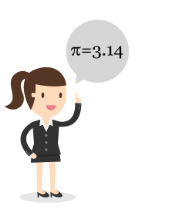# GMAT Quant: Arithmetic – DecimalsDecimals is a topic that was introduced in the primary school and with time it only got to be tough in concept but never left the math students alone. This article on
GMAT Arithmetic – Decimal – will help you in understanding that decimal is not as scary as it seems to be.

For example – Decimal Figures – 0.12, 1.85, 36.73, 99.99

## Decimal Fractions

Any fraction with denominators of the power of 10, is known as decimal fractions.

For example – $\frac{1}{100}$

This figures implies that it is equivalent to 1 hundredth

Hence, $\frac{1}{100}$ = 0.01

## Decimal to Fraction Conversion

To convert 0.85 to a fraction

Step 1 – Divide the number by 1

$\frac{0.85}{1}$

Step 2 – As there are 2 digits after the decimal point, you need to multiply both the numerator and denominator of the figure with 100 (as it has two zeros)

Hence, $\frac{0.85}{1} = \frac{0.85}{1} \times \frac{100}{100} = \frac{85}{100}$

Step 3 – Simplify the Fraction

$\frac{85}{100} = \frac{17}{20}$

## Shortcut Decimals

It is recommended to memorize the below mentioned fractions and their respective decimal figures.

 Fraction Decimal $\frac{1}{2}$ 0.5 $\frac{1}{3}$ 0.3333333333333333… $\frac{2}{3}$ 0.6666666666666666… $\frac{1}{4}$ 0.25 $\frac{3}{4}$ 0.75 $\frac{1}{5}$ 0.2 $\frac{1}{6}$ 0.16666666666666666… $\frac{5}{6}$ 0.83333333333333333… $\frac{1}{8}$ 0.25 $\frac{1}{9}$ 0.11111111111111111… $\frac{1}{11}$ 0.090909090909090909…

Let’s solve some examples and understand its basic concepts.

1. Solve $\left ( \frac{0.9984}{0.96} – 1 \right )$

Solution:

$0.9984 = 1 – 0.0016$

$= 1 – (0.04)^{2}$

$= (1 + 0.04)(1 – 0.04)$

As, $a^{2} – b^{2} = (a + b)(a – b)$

Now, the equation can be written as,

$\frac{(1 – 0.04)(1 + 0.04)}{(1 – 0.04)} – 1$

$(1 + 0.04) – 1$

$= 0.04$

Click for basic Arithmetic Formulas for GMAT.

BYJU’S will be glad to help you in your GMAT preparation journey. You can ask for any assistance related to GMAT and MBA from us by calling us at +918884544444. You can write to us at gmat@byjus.com.